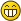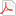ZerationMphLeeLong Time FellowPosts: 375 Threads: 30 Joined: May 2013 06/30/2022, 11:32 PM Quote:Then, why not make a zerated to the a equal a plus 3 halves as a compromise? Because that doensn't make any sense. I know that to someone who is not familiar with the material, all the disccussion about zeration sounds meaningless and arbitrary, but it isn't. I encourage you to read the literature about that. Rubtsov and Romerio gave great motivation and heuristics in their papers. There is also Cesco Reale's article, and many rich threads on this forum. Look for them. The keyword here is mother law. MSE MphLee Mother Law $(\sigma+1)0=\sigma (\sigma+1)$ S Law $\bigcirc_f^{\lambda}\square_f^{\lambda^+}(g)=\square_g^{\lambda}\bigcirc_g^{\lambda^+}(f)$CatullusFellowPosts: 213 Threads: 47 Joined: Jun 2022 06/30/2022, 11:37 PM (This post was last modified: 07/01/2022, 09:37 AM by Catullus.) (06/30/2022, 11:32 PM)MphLee Wrote: Quote:Then, why not make a zerated to the a equal a plus 3 halves as a compromise? Because that doensn't make any sense. I know that to someone who is not familiar with the material, all the disccussion about zeration sounds meaningless and arbitrary, but it isn't. I encourage you to read the literature about that. Rubtsov and Romerio gave great motivation and heuristics in their papers. There is also Cesco Reale's article, and many rich threads on this forum. Look for them. The keyword here is mother law.The Mother Law would make a°a=a+1. The Grand-Mother Law would make a°a=a+2. Why not make a°a=a+1.5, As a compromise zeration? Please remember to stay hydrated. ฅ(ﾐ⚈ ﻌ ⚈ﾐ)ฅ Sincerely: Catullus /ᐠ_ ꞈ _ᐟ\MphLeeLong Time FellowPosts: 375 Threads: 30 Joined: May 2013 06/30/2022, 11:47 PM Again, because that doesn't make any sense. If you do that you are imposing the failure of both the laws, something that, per se, is a folish move if you don't come up with a better law. And the purpose of the laws is to add constraints to the functional equations in order to fully determine solutions to of hyperoperations where the zeroth-rank is a total function. Again, you need to read more literature and put some effort. MSE MphLee Mother Law $(\sigma+1)0=\sigma (\sigma+1)$ S Law $\bigcirc_f^{\lambda}\square_f^{\lambda^+}(g)=\square_g^{\lambda}\bigcirc_g^{\lambda^+}(f)$MphLeeLong Time FellowPosts: 375 Threads: 30 Joined: May 2013 12/28/2022, 01:28 PM I want to point to this other thread where a novel possible definition for operation preceding addition is proposed by user Danteman163. It seems to me that this idea was never been described before. https://math.eretrandre.org/tetrationfor...32#pid9332 MSE MphLee Mother Law $(\sigma+1)0=\sigma (\sigma+1)$ S Law $\bigcirc_f^{\lambda}\square_f^{\lambda^+}(g)=\square_g^{\lambda}\bigcirc_g^{\lambda^+}(f)$JmsNxnUltimate FellowPosts: 1,214 Threads: 126 Joined: Dec 2010 12/30/2022, 02:45 AM (This post was last modified: 12/30/2022, 03:48 AM by JmsNxn.) (12/28/2022, 01:28 PM)MphLee Wrote: I want to point to this other thread where a novel possible definition for operation preceding addition is proposed by user Danteman163. It seems to me that this idea was never been described before. https://math.eretrandre.org/tetrationfor...32#pid9332 Hmm I actually really like that. Especially his description. Is that a viable operator though...? Personally I still like the $\Gamma$ function interpretation. $a \langle 0 \rangle b = a + b\\$ Then: \begin{align} a \langle -1 \rangle b &= a +1\\ a \langle -2 \rangle b &= a+1\\ a \langle -3 \rangle b &= a+1\\ &\vdots \end{align} But, there's singular behaviour at each of these points. And that small perturbations in the hyper operational indexes are wild and destructive. Additionally, the action of going back becomes idempotent. There isn't a 1-1 in the mapping $\langle q \rangle \to \langle q-1\rangle$. But this only happens at the singular points at $-1,-2,-3,...$. You need local data near $q \approx -2$ to pull back to addition again. Despite $q =-2$ alone making $q=-1$ addition when you try to naively map forward $\langle q \rangle \to \langle q+1\rangle$. Where doing this naively just iterates the predecessor. Doing it more difficultly requires iterating a neighborhood of the predecessor. I apologize. I like this idea from zeration. But honestly. It should be successorship all the way down, but at each singularity, there is different behaviour in a neighborhood of each successorship.MphLeeLong Time FellowPosts: 375 Threads: 30 Joined: May 2013 01/05/2023, 08:53 PM I agree, in fact I'm working on Goodstein-like solutions on my quest for naturality. But there can be a non-trivial zerations zoo of solutions. And this thread is all about that alternative exotic landscape. One day we will be able to appreciate better its taste and meaning I believe, eg. its relationship to tropical math. MSE MphLee Mother Law $(\sigma+1)0=\sigma (\sigma+1)$ S Law $\bigcirc_f^{\lambda}\square_f^{\lambda^+}(g)=\square_g^{\lambda}\bigcirc_g^{\lambda^+}(f)$JmsNxnUltimate FellowPosts: 1,214 Threads: 126 Joined: Dec 2010 01/10/2023, 05:59 AM (01/05/2023, 08:53 PM)MphLee Wrote: I agree, in fact I'm working on Goodstein-like solutions on my quest for naturality. But there can be a non-trivial zerations zoo of solutions. And this thread is all about that alternative exotic landscape. One day we will be able to appreciate better its taste and meaning I believe, eg. its relationship to tropical math. Oh yes! For sure Mphlee. There's a whole zeration zoo. Same way there is a zoo of "interpolations of the factorial". But they're all garbage other than $\Gamma$, lmao.NatsugouNewbiePosts: 1 Threads: 0 Joined: Oct 2023 10/17/2023, 06:54 AM (This post was last modified: 10/17/2023, 10:05 AM by Natsugou. Edit Reason: it didn't make sense. ) (01/05/2023, 08:53 PM)MphLee Wrote: I agree, in fact I'm working on Goodstein-like solutions on my quest for naturality. But there can be a non-trivial zerations zoo of solutions. And this thread is all about that alternative exotic landscape. One day we will be able to appreciate better its taste and meaning I believe, eg. its relationship to tropical math. Hello MphLee! I'm interested in negative-rank hyperoperations, and I posted this on the googology fandom of japan. I tried to solve the equation for bi-infinite sequence $(\star_r: \mathbb{Z}_{\geq 2} \times \mathbb{Z}_{\geq 2} \to \mathbb{Z}_{\geq 2})_{r = -\infty}^{\infty}$ as follows: $\forall m \forall r \forall n; m \star_{r+1} (n+1) = m \star_r (m \star_{r+1} n) \land m \star_{r+1} 2 = m \star_r m \land m \star_1 n = m+n.$ I proofed the existence of solutions and found one, $\star_0 = \heartsuit$ such that $m \heartsuit n = \begin{cases} n & \text{if}\; 2 \leq n \leq m-1 \\ n+2 & \text{if}\; n = m \\ n & \text{if}\; n = m+1 \\ n+1 & \text{if}\; n \geq m+2. \end{cases}$ Then all negative elements are uniquely determined as $m \star_{-1} n = \left\{ \begin{array}{lll} & & \text{if $m = 2$ and} \\ n+1 & \leq 1 & n \leq 0 \\ n+1 & = 2 & n = 1 \\ n+2 & = 4 & n = 2 \\ n+2 & = 5 & n = 3 \\ n-1 & = 3 & n = 4 \\ n+1 & \geq 6 & n \geq 5 \\ & & \text{if $m = 3$ and} \\ n+1 & \leq 1 & n \leq 0 \\ n+2 & = 3 & n = 1 \\ n+3 & = 5 & n = 2 \\ n-1 & = 2 & n = 3 \\ n+2 & = 6 & n = 4 \\ n-1 & = 4 & n = 5 \\ n+1 & \geq 7 & n \geq 6 \\ & & \text{if $m \geq 4$ and} \\ n+1 & \leq 1 & n \leq 0 \\ m & = m & n = 1 \\ n+1 & \geq 3, \leq m-1 & 2 \leq n \leq m-2 \\ n+3 & = m+2 & n = m-1 \\ 2 & = 2 & n = m \\ n+2 & = m+3 & n = m+1 \\ n-1 & = m+1 & n = m+2 \\ n+1 & \geq m+4 & n \geq m+3 \end{array} \right.$ $m \star_{-2} n = \left\{ \begin{array}{lll} & & \text{if $m = 2$ and} \\ n+1 & \leq 1 & n \leq 0 \\ n+1 & = 2 & n = 1 \\ n+2 & = 4 & n = 2 \\ n+3 & = 6 & n = 3 \\ n+1 & = 5 & n = 4 \\ n-2 & = 3 & n = 5 \\ n+1 & \geq 7 & n \geq 6 \\ & & \text{if $m = 3$ and} \\ n+1 & \leq 1 & n \leq 0 \\ n+2 & = 3 & n = 1 \\ n+4 & = 6 & n = 2 \\ n+2 & = 5 & n = 3 \\ n+3 & = 7 & n = 4 \\ n-3 & = 2 & n = 5 \\ n-2 & = 4 & n = 6 \\ n+1 & \geq 8 & n \geq 7 \\ & & \text{if $m = 4$ and} \\ n+1 & \leq 1 & n \leq 0 \\ n+3 & = 4 & n = 1 \\ n+5 & = 7 & n = 2 \\ n+3 & = 6 & n = 3 \\ n-1 & = 3 & n = 4 \\ n+3 & = 8 & n = 5 \\ n-4 & = 2 & n = 6 \\ n-2 & = 5 & n = 7 \\ n+1 & \geq 9 & n \geq 8 \\ & & \text{if $m \geq 5$ and} \\ n+1 & \leq 1 & n \leq 0 \\ m & = m & n = 1 \\ m+3 & = m+3 & n = 2 \\ n+1 & \geq 4, \leq m-1 & 3 \leq n \leq m-2 \\ n+3 & = m+2 & n = m-1 \\ 3 & = 3 & n = m \\ n+3 & = m+4 & n = m+1 \\ 2 & = 2 & n = m+2 \\ n-2 & = m+1 & n = m+3 \\ n+1 & \geq m+5 & n \geq m+4 \end{array} \right.$ ... If the domain and codomain are limited to $\mathbb{Z}_{\geq 2}$, we can interpolate the hyperoperation sequence. Attached Filesruikaeigo.pdf (Size: 431.59 KB / Downloads: 29) « Next Oldest | Next Newest »

 Possibly Related Threads… Thread Author Replies Views Last Post More literature on zeration mathexploreryeah 1 368 10/09/2023, 11:25 PM Last Post: leon Zeration reconsidered using plusation. tommy1729 1 6,925 10/23/2015, 03:39 PM Last Post: MphLee Is this close to zeration ? tommy1729 0 4,763 03/30/2015, 11:34 PM Last Post: tommy1729  4th Zeration from base change pentation tommy1729 5 14,485 03/29/2015, 05:47 PM Last Post: tommy1729  New zeration and matrix log ? tommy1729 1 7,382 03/24/2015, 07:07 AM Last Post: marraco Zeration = inconsistant ? tommy1729 20 54,415 10/05/2014, 03:36 PM Last Post: MphLee

Users browsing this thread: 1 Guest(s)